Home | Gadget | Fuzzy | Lecturer  | Power  | Transceiver Find more type here... Integrator by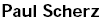Solve for Vout in terms of Vin. If you need to process signal with math function integrator, learn this explanation.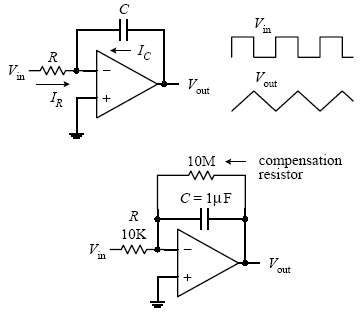Because you have feedback, and because V+ = 0 V, you can say that V− is 0 V as well (rule 4).Now that you know V−, solve for IR and IC so that you can come up with an expression relating Vout with Vin. Since no current enters the input of an op amp (rule 3), the  displacement current IC through the capacitor and the current IR through the resistor must be related by IR + IC = 0.To find IR, use Ohm’s law: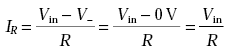IC is found by using the displacement current relation: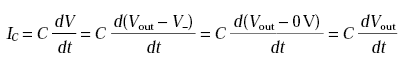Placing these values of IC and IR into IR + IC = 0 and rearranging, you get the answer: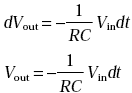Such a circuit is called an integrator; the input signal is integrated at the output. Now, one problem with the first circuit is that the output tends to drift, even with the input grounded, due to non ideal characteristics of real op amps such as voltage offsets and bias current. A large resistor placed across the capacitor can provide dc feedback for stable biasing. Also, a compensation resistor may be needed between the non inverting terminal and ground to correct voltage offset errors caused by input bias currents. The size of this resistor should be equal to the parallel resistance of the input resistor and the feedback compensation resistor. This material comes from any source reference and the copyright is own the author him self not this sites Copyright 2009 (c) technosains.com  All Rights Reserved